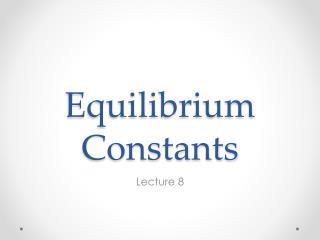DownloadDownload PresentationEquilibrium Constants

# Equilibrium Constants

Télécharger la présentation## Equilibrium Constants

- - - - - - - - - - - - - - - - - - - - - - - - - - - E N D - - - - - - - - - - - - - - - - - - - - - - - - - - -
##### Presentation Transcript

1. Equilibrium Constants Lecture 8

2. The Equilibrium Constant • Consider the reaction aA + bB = cC + dD • The Free Energy change of reaction is: ∆G = cµc + dµd – aµa – bµb • At equilibrium: • Expanding the right side • or • We define the right term as the equilibrium constant:

3. Free Energy and the Equilibrium Constant • Since: • then • and • Note of caution: our thermodynamic parameters are additive, but because of the exponential relation between the equilibrium constant and free energy, equilibrium constants are multiplicative.

4. Manipulating Equilibrium Constants • Suppose we want to know the equilibrium constant for a reaction that can be written as the sum of two reactions, • e.g., we can sum • to yield • The equilibrium constant of the net reaction would be the product of the equilibrium constants of the individual reactions. • For this reason and because equilibrium constants can be very large or very small numbers, it is often convenient to work with logs of equilibrium constants: pK = - log K • (we can then sum the pK’s).

5. Apparent Equilibrium Constants and Distribution Coefficient • In practice, other kinds of equilibrium constants are used based on concentrations rather than activities. • Distribution Coefficient • Apparent Equilibrium Constant

6. Other Forms • A ‘solubility constant’ is an equilibrium constant. For example: • Since the activity of NaCl in halite = 1, then • Henry’s Law constants for describing solubility of gases in solution (e.g., CO2 in water). • Since Pi = hiXi

7. Law of Mass Action • Important to remember our equation describes the equilibrium condition. At non-equilibrium conditions it is called the reaction quotient, Q. • Written for the reaction H2CO3 = HCO3- + H+ • We can see that addition of H+ will drive the reaction to the left. • “Changing the concentration of one species in a reaction in a system at equilibrium will cause a reaction in a direction that minimizes that change”.

8. Le Chatelier’s Principle • We can generalize this to pressure and temperature: dG = VdP - SdT • An increase in pressure will drive a reaction in a direction such as to decrease volume • An increase in temperature will drive a reaction in a direction such as to increase entropy. • “When perturbed, a system reacts to minimize the effects of perturbation.”

9. Temperature and Pressure Dependence • Since ∆G˚ = ∆H˚ - T∆S˚ and ∆G˚ = -RT lnK then • Temperature and pressure dependencies found by taking derivatives of this equation with respect to T and P.

10. Oxidation and Reduction Oxidation refers to processes in which atoms gain or loss electrons, e.g., Fe2+ Fe3+

11. Valence and Redox • We define valence as the charge an atom acquires when it is dissolved in solution. • Conventions • Valence of all elements in pure form is 0. • Sum of valences much equal actual charge of species • Valence of hydrogen is +1 except in metal hydrides when it is -1 • Valence of O is -2 except in peroxides when it is -1. • Elements generally function as either electron donors or acceptors. • Metals in 0 valence state are electron donors (become positively charged) • Oxygen is the most common electron acceptor (hence the term oxidation) • Redox • A reduced state can be thought of as one is which the availability of electrons is high • An oxidized state is one in which the availability of electrons is low.